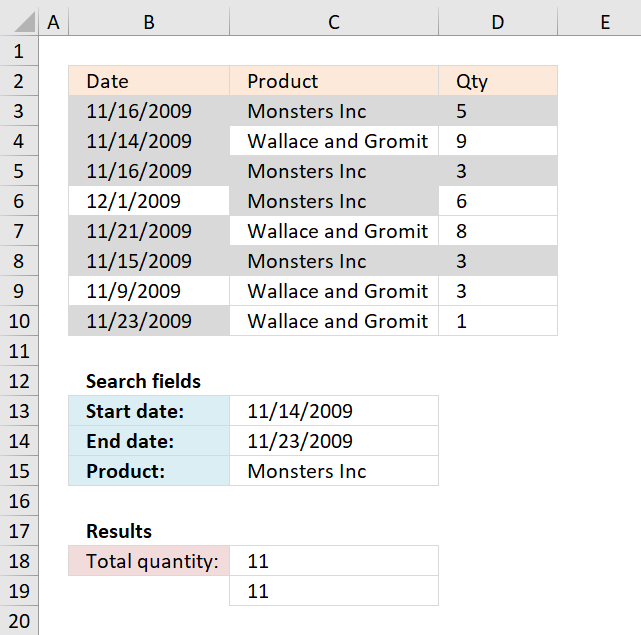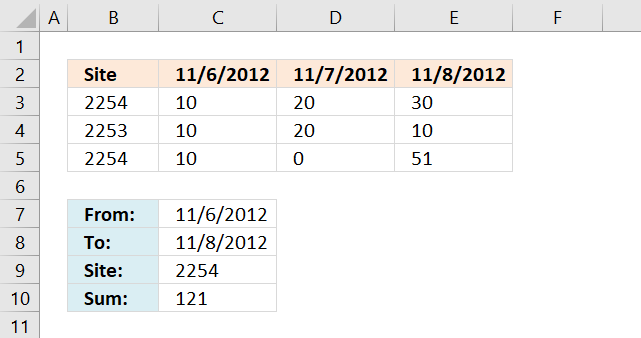Author: Oscar Cronquist Article last updated on December 07, 2018In this post, I will provide a formula to sum values in column (Qty) where a column (Date) meets two date criteria and an additional criterion in an adjacent column (Product).

I have colored the cells in column Qty that meet all criteria.

Excel formula in C18:

=SUMIFS(D3:D10, C3:C10, "="&C15, B3:B10, "<="&C14, B3:B10, ">="&C13) + ENTER

The SUMIFS function adds numbers based on a condition or criteria and returns a total.

SUMIFS(sum_range, criteria_range1criteria1[criteria_range2], [criteria2], ...)

The sum_range contains the numbers to be added: D3:D10

criteria_range1 (C3:C10) is the cell range that the criteria1 ("="&C15) will be applied to.

criteria_range2 (B3:B10) is the cell range (dates) that the criteria2 ("<="&C14) will be applied to.

criteria_range3 (B3:B10) is the cell range (dates) that the criteria3 (">="&C13) will be applied to.

SUMIFS(D3:D10, C3:C10, "="&C15, B3:B10, "<="&C14, B3:B10, ">="&C13)

Alternative  formula in C19:

=SUMPRODUCT(--(B3:B10<=C14), --(B3:B10>=C13), --(C3:C10=C15), D3:D10) + ENTER

The SUMIFS function was introduced in Excel 2007, the SUMPRODUCT function works in all Excel versions.

Recommended post

Recommended articles

Sum cells with check boxes
I will now demonstrate with the following table how to add check-boxes and sum enabled check-boxes using a formula. Add […]

Recommended articles

Sum number based on corresponding unique value
The formula in cell E14 adds a number from column C if the corresponding value in column B is unique […]

### Get excel file for this tutorial

Sum values between two dates with criteria.xls
(Excel 97-2003 Workbook *.xls)

Functions in formulas above

Recommended articles

How to use the SUM function
The SUM function in Excel allows you to add values, the function returns the sum in the cell it is […]

Recommended articles

How to use the SUMPRODUCT function
The SUMPRODUCT function calculates the product of corresponding values and then returns the sum of each multiplication.

Recommended articles

Site 6-Nov-12 7-Nov-12 8-Nov-12
2254 10 20 30
2253 10 20 10
2254 10 0 51
Criteria: required 2254 sum of values b/w my specified date let say from 6-8 Nov.Formula in cell C10:

=SUMPRODUCT(((\$C\$7<=\$C\$2:\$E\$2)*(\$C\$8>=\$C\$2:\$E\$2))*(\$B\$3:\$B\$5=\$C\$9)*\$C\$3:\$E\$5)

Farhan.xlsx